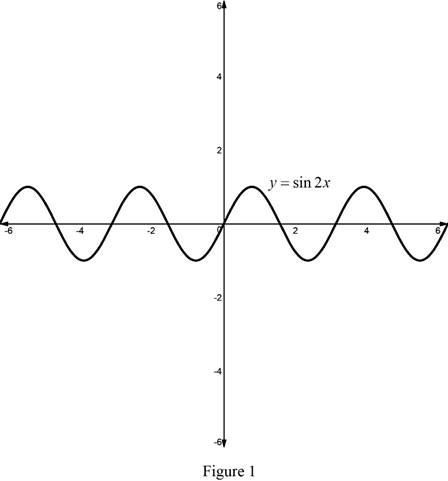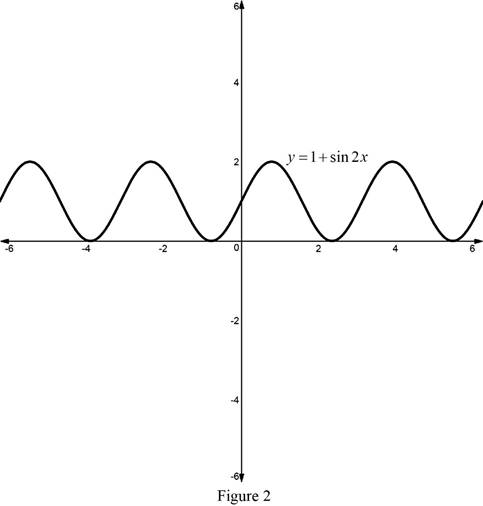# The graph of the function y = 1 + sin 2 x without using a calculator.### Single Variable Calculus: Concepts...

4th Edition
James Stewart
Publisher: Cengage Learning
ISBN: 9781337687805### Single Variable Calculus: Concepts...

4th Edition
James Stewart
Publisher: Cengage Learning
ISBN: 9781337687805

#### Solutions

Chapter T, Problem 9DDT
To determine

## To sketch: The graph of the function y=1+sin2x without using a calculator.

Expert Solution

### Explanation of Solution

Compute a table for various values of x and then use the value of the function to sketch a smooth curve of y=sin2x.

 x −π2 −π4 0 π4 π2 y 0 −1 0 1 0

The graph for the given function y=sin2x is shown below in Figure 1.According to the transaltion and reflection of the function the graph of the function y=1+sin2x is obtained if the graph of y=sin2x is translated upward by 1 units.

The graph for the given function y=1+sin2x is shown below in Figure 2.From Figure 2 , it is observed that the graph of the function y=1+sin2x is obtained if the graph of the function y=sin2x is translated right by 1 units and upward by 1 units.

### Have a homework question?

Subscribe to bartleby learn! Ask subject matter experts 30 homework questions each month. Plus, you’ll have access to millions of step-by-step textbook answers!